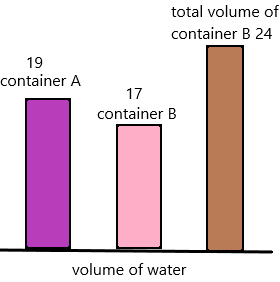# Math in Focus Grade 2 Chapter 9 Practice 4 Answer Key Real-World Problems: Volume

Practice the problems of Math in Focus Grade 2 Workbook Answer Key Chapter 9 Practice 4 Real-World Problems: Volume to score better marks in the exam.

## Math in Focus Grade 2 Chapter 9 Practice 4 Answer Key Real-World Problems: Volume

Solve.

Question 1.
Mrs. White brings 70 liters of juice to the school picnic. After the picnic, 12 liters of juice are left. How many liters of juice does everyone drink at the picnic? They drink ____ litres of juice at the picnic.
Explanation:
The total number of litres White brings juice to the picnic=70
The number of litres of juice is left=12
We need to calculate the number of litres of juice does everyone drink. Assume it as X.
To find the result, subtract the total amount of juice and the juice that is left.
X=70-12
X=56.
Therefore, 58 litres of juice had drunk at the picnic.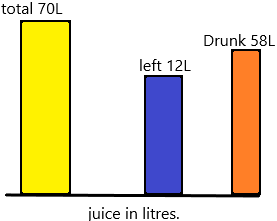Question 2.
A fish tank contains 12 liters of water. Another fish tank contains 7 liters of water. What is the total volume of water in the two fish tanks?
The total volume of water in the two tanks is __ litres.
Explanation:
The number of litres is present in one fish tank=12
The number of litres is present in another tank=7.
The total volume of water in two tanks=X
X=12+7
X=19.
Therefore, there are 19 litres in the two tanks.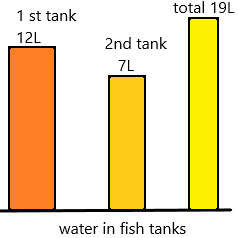Solve.

Question 3.
Sylvia fills two containers of the same size with water. She fills Container A with 5 liters of water. Then she fills Container B with 3 more liters of water than Container A. What is the total volume of water in both containers?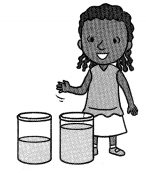There are ___ liters of water in both containers.
Explanation:
The number of litres she fills container A=5
The number of litres she fills container B=5+3=8 (she fills 3 litres more than container A)
The total volume of water in both the containers=X
add both the volumes to get the total volume in containers.
X=8+5
X=13
Therefore, 13 litres are present in both containers.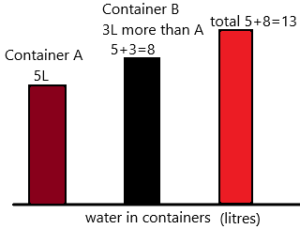Question 4.
A barrel has 60 liters of rainwater. Jan uses 17 liters to water her flower garden. She uses another 15 liters to water her vegetable garden. How much rainwater is left in the barrel?
There are ___ liters of rainwater left in the barrel.
Explanation:
The total number of litres of rainwater a barrel has=60
The number of litres she uses for her flower garden=17
The number of litres she uses for vegetable garden=15
The total number of litres she used=15+17=32.
We need to calculate the number of rainwater left in the barrel:
The rainwater left=X
subtract the total rainwater and rainwater used to get the rainwater left in the barrel.
X=60-32
X=28
Therefore, 28 litres are left in the barrel.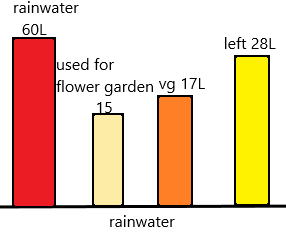Solve.

Question 5.
Container A has 18 litres of water. Container B has 5 liters of water more than Container A. Container C has 16 liters of water less than Container B. What is the volume of water in Container C?
There are ____ litres of water in Container C.
Explanation:
The number of litres of water container A have=18
The number of litres of water container B have=18+5=23 (5 litres more than container A).
The number of litres of water container C have=23-16=7 (16 litres less than container B).
Therefore, the volume of water in container C is 7 litres.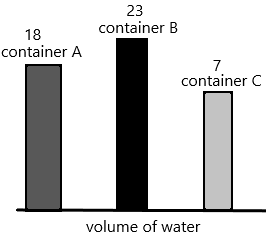Question 6.
Container A has 17 litres of water. It has 2 more litres of water than Container B Jasmine pours another 7 liters of water into Container B. What is the volume of water in Container B?
There are ___ litres of water in container B.